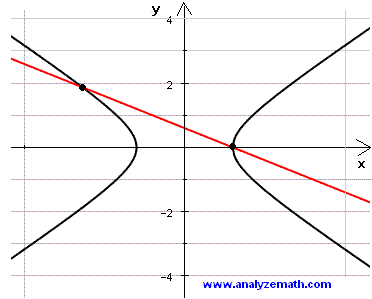# Points of Intersection of a Hyperbola and a Line

This is tutorial on finding the points of intersection of a hyperbola and a line given by their equations.

Example 1: Find the points of intersection of a hyperbola and a line given by their equations as follows:

x2 / 9 - y2 = 1
x + 5y = 3

Solution to Example 1:

• Solve the equation of the line for x to obtain:
x = 3 - 5y
• We now substitute x by 3 - 5y into the equation of the hyperbola to obtain
(3 - 5y)2 / 9 - y2 = 1
• Expand and group like terms and rewrite the equation as
16y2 -30y = 0
• Solve the quadratic equation for y to obtain two solutions
y = 0 and y = 15/8
• We now substitute the values of y already obtained into x = 3 - 5y to obtain
for y = 0, x = 3 and for y = 15/8, x = -51/8
• The 2 points of intersection of the the hyperbola and the line are
( 3 , 0) ; ( -51/8 , 15/8)

Shown below is the graph of a hyperbola, a line and their points of intersection.## More References and Links to Hyperbolas

Equation of Hyperbola- Graphing Problems.hyperbola equation
Find the Points of Intersection of Two Hyperbolas
Points of Intersection of a Hyperbola and a Line